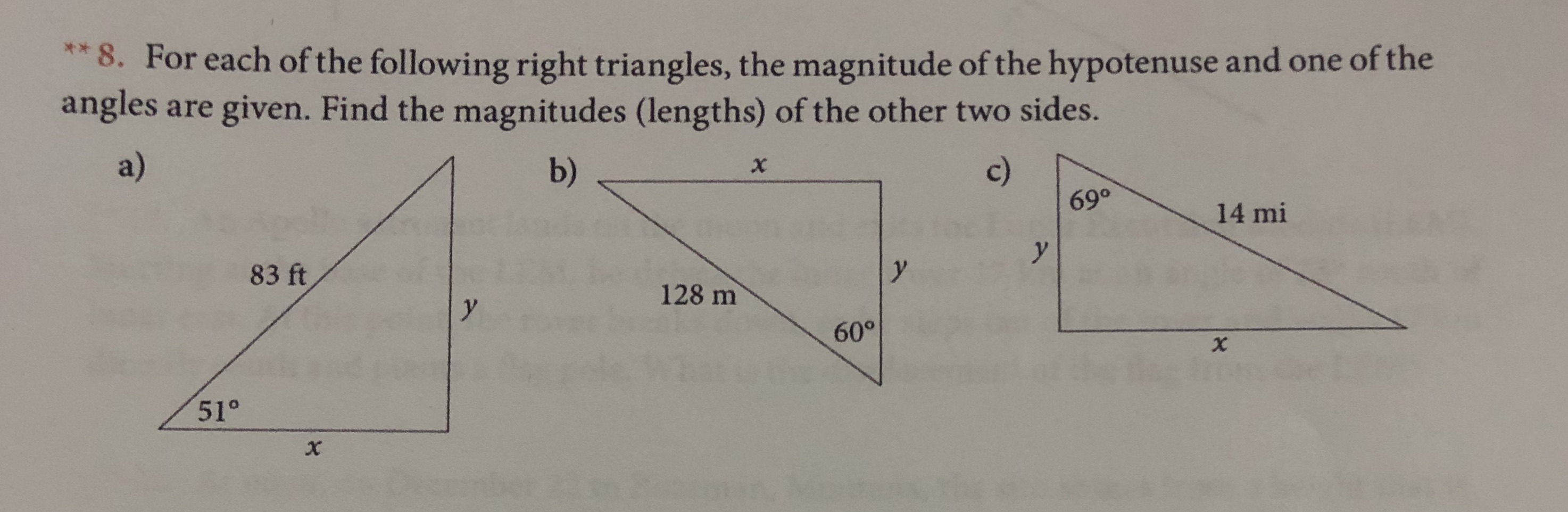**8. For each of the following right triangles, the magnitude of the hypotenuse and one of theanglesare given. Find the magnitudes (lengths) of the other two sides.a)c)b)X14 miyy83 ft128 my60°51°X

Questionhelp_outlineImage Transcriptionclose**8. For each of the following right triangles, the magnitude of the hypotenuse and one of the angles are given. Find the magnitudes (lengths) of the other two sides. a) c) b) X 14 mi y y 83 ft 128 m y 60° 51° X fullscreen
Step 1

Part a.

Write the expression for magnitude of the hypotenuse of a triangle.

Step 2

Write the expression for direction of two vectors x and y.

Step 3

Substitute the corresponding values i...

Want to see the full answer?

See Solution

Want to see this answer and more?

Our solutions are written by experts, many with advanced degrees, and available 24/7

See Solution
Tagged in

Vectors and Scalars